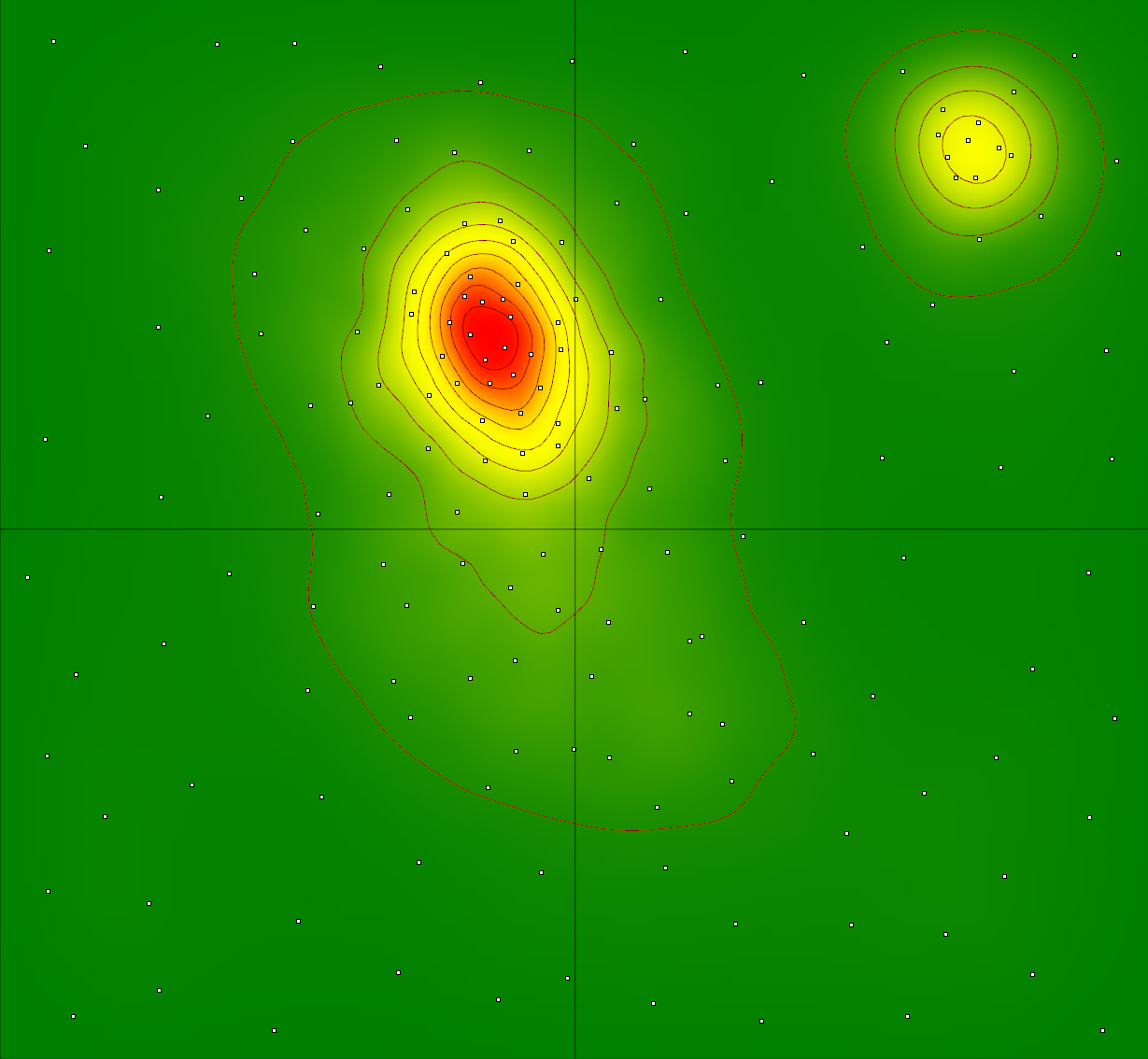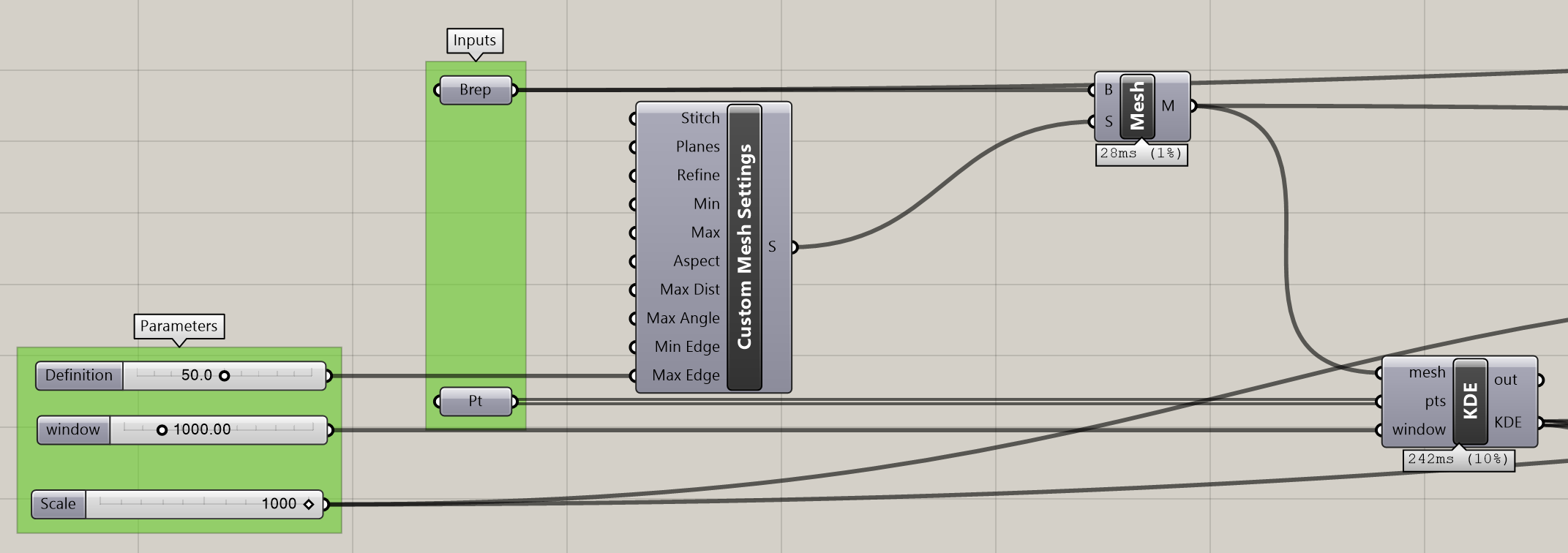## Kernel Density Estimation

Category : Code, Research

Following the presentation of the article Measuring Urban Renewal: a dual kernel density estimation to assess the intensity of building renovation at the 4th International Symposium Formal Methods in Architecture 2018 in Porto, I will share some of the tools I developed to perform Kernel Density Estimation on Grasshopper for Rhino. Kernel Density Estimation (KDE) is a method of estimating the smooth probability density function of an underlying random variable. The estimation is performed using a finite sample of data. Matthew Conlen explains in a simple and interactive way how the choice of kernel function and window influences the shape of the estimation function. For a more in-depth introduction, Yen-Chi Chen wrote a tutorial on Kernel Density Estimation and Recent Advances available at https://arxiv.org/abs/1704.03924.A kernel density estimation

### Grasshopper script

The image above is an example of a KDE. To produce an estimate in grasshopper two inputs are needed: an analysis surface and points. The script calculates an estimate value for each vertex of the mesh. The definition of the estimate is determined by the number of vertices of the mesh. Consequently, the more vertices the more values are estimated, which can quickly translate to high computation times. So, the definition should be set to a large value and reduced to smaller values to improve the quality of the mesh. The second parameter is the window width. It is the smoothing factor of the estimate and determines the radius of the kernel function. Larger values will smooth the surface more, while smaller values will provide a “bumpier” estimate. The scale parameter is optional and is useful for 3d visualizations of the estimate.### C# code

This code is designed for the C# component in Grasshopper. The first section should be placed on the first space and the second on the last in the C# editor of grasshopper. The header (private void…) is provided for guidance on the expected inputs and outputs of the script that should be added on the grasshopper C# component UI as in the picture above. The inputs must be named correctly, the type hints must be set to the correct object type (mesh, Point3d, double respectively). The point input must be set to list access also.

```private void RunScript(Mesh mesh, List&lt;Point3d&gt; pts, double window, ref object KDE)
{
//beginning of the first section
var rtnlist = new List<double>();
for(int i = 0; i <; mesh.Vertices.Count; i++)
{
double tempval = 0.0;
double kde = 0.0;
Point3d v = new Point3d(mesh.Vertices[i]);
for(int j = pts.Count - 1; j > 0; j--)
{
var pt = pts[j];
Vector3d vt = new Vector3d(v - pt);
tempval += kernel(vt / window);
}
kde = 1 / (pts.Count * window * window) * tempval;
}
KDE = rtnlist;
//end of first section
}

//beginning of the second section
public double kernel(Vector3d input)
{
//Epanechnikov Kernel
double k = Vector3d.Multiply(input, input);
if ( k < 1)
{
return 3 * (1 / Math.PI) * (1 - k) * (1 - k);
}else{
return 0;
}
}```

The last step involves colouring the mesh within the scale of the estimate. Optionally, it is possible to scale the mesh to better represent the estimate in 3D.